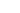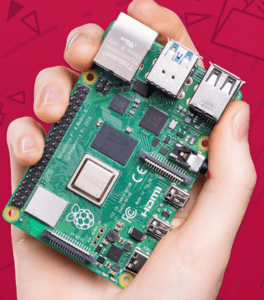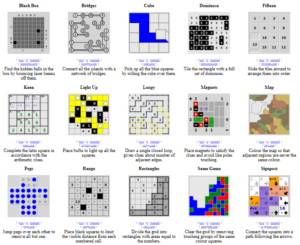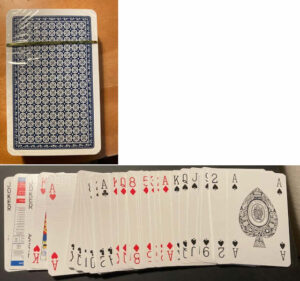### Category: C## Restarting the Raspberry PI C Games tutorialsI believe that the Raspberry PI, especially the 4B is a great and very low cost machine for not only running games but for developing them as well. Of course, if you have a PC, Linux or Mac then you can use that as a development machine but if you haven’t, it costs less than £100 (when you count the system, case, cables, SD-Card) to get up and running.

So I’m reworking my original eBook for the Raspberry Pi, using software running on the Pi and developing a 2nd ebook. Along the way I’ll publish longer excerpts from it here. Probably one a week.## More on Shuffledness

So I’ve updated my shuffledness calculation. This is a bit of a work in progress so probably isn’t the last version.  One of the things I’m interested in is what is the optimal number of swaps to get a good shuffled deck of cards. The shuffle algorithm picks two random indexes in the array 0-51 and then swaps the contents.

Obviously doing this 10 times would only shuffle at most 20 cards and probably fewer as there’s no checks for repeats. The only check is that both indexes (indices?) are not the same. So I want to experiment and see what an optimal value for numTries should be..

``````void ShuffleDeck(int numtries) {
int firstIndex, secondIndex;
for (int i = 0; i < numtries; i++) {
do {
firstIndex = rand() % 52;
secondIndex = rand() % 52;
}
while (firstIndex == secondIndex);
int value = deck[firstIndex];
deck[firstIndex] = deck[secondIndex];
deck[secondIndex] = value;
}
}``````

Anyway, I’ve added a CalcDistance() function. This looks at each card and calculates how far it has moved from it’s original position.  It then divides this by the maximum distance it could have moved to get a move “factor”. These are summed up and divided by 52 to give an average move factor. That’s your CalcDistance() function.

``````float CalcDistance() {
float distance = 0.0f;
for (int i = 0; i < 52; i++) {
float distanceDiv = (i < 26) ? 51 - i : i-1;
//printf("%i: %3.0f\n", i, distanceDiv);
distance += (abs(deck[i] - i) / distanceDiv);
}
return distance/52.0f;
}``````

The only thing odd about this function is the distanceDiv value which starts at 51, decreases to 25 then increases to 50. The printf let me check that was correct.

This is the current state of the program which I’ve listed fully below. Enjoy! It’s 63 lines long.

``````// shuffledness.c : This measures how shuffled a deck of cards is
// cards are hjeld as values 0-51 in a 52 int aaray.

#include <time.h>
#include <stdio.h>
#include <stdlib.h>

int deck;
time_t t;

// Order the cards 0-51. 0 = Acew Hards,13 = Ace Clubs,26 = Ace of Diamonds and 51 = King of Spades
void InitDeck() {
for (int i = 0; i < 52; i++) {
deck[i] = i;
}
}

// Works on glovbal array deck and calculates a value for how displayed each card is
float CalcDisorder() {
int total=0;
for (int i = 1; i < 52; i++) {
total += abs(deck[i] - deck[i - 1]);
}
printf("Total = %d\n", total);
}

// Sum up distance card moved/max distance
float CalcDistance() {
float distance = 0.0f;
for (int i = 0; i < 52; i++) {
float distanceDiv = (i < 26) ? 51 - i : i-1;
//printf("%i: %3.0f\n", i, distanceDiv);
distance += (abs(deck[i] - i) / distanceDiv);
}
return distance/52.0f;
}

// Shuffle a deck by swapping two random cards a specified number of times
void ShuffleDeck(int numtries) {
int firstIndex, secondIndex;
for (int i = 0; i < numtries; i++) {
do {
firstIndex = rand() % 52;
secondIndex = rand() % 52;
}
while (firstIndex == secondIndex);
int value = deck[firstIndex];
deck[firstIndex] = deck[secondIndex];
deck[secondIndex] = value;
}
}

int main() {
/* Intializes random number generator */
srand((unsigned)time(&t));
for (int i = 0; i < 25; i++) {
InitDeck();
ShuffleDeck(1000);
printf("CalcDisorder() ==%f x %f = %f\n", CalcDisorder(),CalcDistance(),(double)CalcDisorder() * CalcDistance());
}
return 0;
}
``````

Because I’m multiplying the two factors (disorder and Distance, the final value is never getting much above 0.35 and I’d prefer it to be in the range 0-0.999. Suggestions welcomed.

.So, if you are new to programming Compiling and linking can seem like magic. The compiler transforms C source code files into obj files. The actual format is different depending on the CPU and Operating system.  Linking is even more magic because it takes a bunch of obj files and produces a single executable.

There’s actual more magic going on than my simplistic explanation. The compiler might be doing optimisation (generally in release mode but not debug). That makes debug compiles faster which is more desirable.

Another blog called Hack the developer has published an article with a lot more detail about compiling and linking. If you want to get a better understanding then this is a petty good piece. It uses Linux, GCC and Clang as examples. It also goes into more depth on the layout of obj files.## 39 Puzzles in CThose of you who have used the Putty utility to connect to a remote computer using SSH might recognise the name chiark. It’s also the home of various puzzles that are coded in C and they’ve made the source code available.  You can read about each puzzle via the online documentation.

The puzzles are one player games and run natively on Unix (GTK), on Windows, and on Mac OS X. As usual I’ve added these to the C code links as well. Puzzles are near enough to games to count! I’ve screen grabbed 15 of them but there are almost 40 in total.

If you like this sort of thing and have an idea for a game, take a look at the devel page. The puzzles are split into three parts, front, middle and back ends. If you want to implement a new front end (SDL2 anyone?) then you just change the front end.## A better random number generator

How’s this for a minimal RNG implementation in C?

``````// *Really* minimal PCG32 code / (c) 2014 M.E. O'Neill / pcg-random.org

typedef struct { uint64_t state;  uint64_t inc; } pcg32_random_t;

uint32_t pcg32_random_r(pcg32_random_t* rng)
{
uint64_t oldstate = rng->state;
rng->state = oldstate * 6364136223846793005ULL + (rng->inc|1);
// Calculate output function (XSH RR), uses old state for max ILP
uint32_t xorshifted = ((oldstate >> 18u) ^ oldstate) >> 27u;
uint32_t rot = oldstate >> 59u;
return (xorshifted >> rot) | (xorshifted << ((-rot) & 31));
}``````

https://www.pcg-random.org/

is a website dedicated to random numbers using their algorithms, The whole site is good but I particularly like the party tricks page where they show that one of their random number generators generates a sequence that contains interesting things like source of a C file, zip files and text from hamlet.## More thoughts on shuffledness

Thinking about what I said, it can’t be just about how far a card moves when shuffled but how much disordering occurs as well. If you shuffled the deck and discovered 7 cards in a sequence like 2,3,4,5,6 of hearts deep into the deck, they would have moved from near the top of the deck (an “ordered deck” is A-K in Hearts, Clubs, Diamonds and Spades) then it probably wasn’t a very good shuffle.

One way to measure “disorder” is to subtract each card’s ordinal value from it’s successor and sum up the absolute value of them . Ordinal value is the value 0-51 where 0 = Ace Hearts, 1 = 2 Hearts and so on. In an ordered deck the sum of all these subtractions will be 51. In a well shuffled deck I would guess it might be something like 1352 (52 x 26). Absolute value is the abs(x) function of x which is always positive if x is negative.  abs(-8) = 8. abs(8) = 8.

So now for shuffledness we have two values. Displacement and disorder. If both are calculated as a value between 0 and 1 then multiplying them together might work as a measure of shuffledness.

Here’s a very simple example of calculating the value for disorder. So far the calculations have never got a total much above 900, and not near 1352. I’ll run a few experiments and see the range of values.

``````// shuffledness.c : This measures how shuffled a deck of cards is
// cards are held as values 0-51 in a 52 int array.

#include <time.h>
#include <stdio.h>
#include <stdlib.h>

int deck;
time_t t;

// Order the cards 0-51. 0 = Acew Hards,13 = Ace Clubs,26 = Ace of Diamonds and 51 = King of Spades
void InitDeck() {
for (int i = 0; i < 52; i++) {
deck[i] = i;
}
}

// Works on glovbal array deck and calculates a value for how displayed each card is
float CalcDisorder() {
int total=0;
for (int i = 1; i < 52; i++) {
total += abs(deck[i] - deck[i - 1]);
}
printf("Total = %d\n", total);
}

// Shuffle a deck by swapping two random cards a specified number of times
void ShuffleDeck(int numtries) {
int firstIndex, secondIndex;
for (int i = 0; i < numtries; i++) {
do {
firstIndex = rand() % 52;
secondIndex = rand() % 52;
}
while (firstIndex == secondIndex);
int value = deck[firstIndex];
deck[firstIndex] = deck[secondIndex];
deck[secondIndex] = value;
}
}

int main() {
/* Intializes random number generator */
srand((unsigned)time(&t));
InitDeck();
ShuffleDeck(1000);
printf("CalcDisorder() == %f\n", CalcDisorder());
return 0;
}``````

That’s about 50 lines long. I’ll work on it and add the displacement calculation and try and get a maximum figure the the disorder calculation.## I’ve updated the C tutorials

I’ve added arrays and structs to the Tutorials page, bringing it up to five so far.  I’ve got 30 or so in total to add and I’ll be adding one or two each week.## A list of open source physics enginesIt’s not uncommon to have 2D games (and 3D) incorporate a physics engine. So when objects move and hit each other they behave realistically. The code that deals with “physical” interaction, objects bouncing or rolling off other objects is usually all parcelled up in a game physics engine.

Doing that means the programmer doesn’t have to worry about objects interacting. Your character moves into a room and knocks a vase; the vase falls over and breaks. Imagine how complex it would be if you had to program all the interactions. Instead, all objects in the room are predefined. As objects move and hit other objects they behave according to the predetermined rules. Balls drop to the floor and bounce. Breakable objects break.

An indie game studio called Tapir Games has put together a pretty comprehensive list of open source game physics engines. There’s even a couple in C though many are programmed in C++, C# and so on.

The picture comes from Chipmunk color match, one of the games using the (C library) Chipmunk physics library.## How to measure how shuffled a deck of cards isI first thought about this when I wrote the program to shuffle  deck of cards using a riffle shuffle. If you are given a deck of cards (or pack of cards as us Brits say), how do you discern just how shuffled the pack is? Can you calculate a numeric value for it say a % ranging from 0 to 100?

I believe it’s possible.  Here’s how.

1. Start with a default pack of cards in perfect sorted order. Out of curiosity I found an unopened deck oif Waddington’s cards and opened it as the photos show. The cards in the pack were arranged in order King, Queen, Jack down to Ace in each of the four suits Heart, Clubs, Diamonds and Spades in that order. Let’s reverse the card rank ordering so a full deck starts with Ace Hearts through to King Hearts, Ace of Clubs to King of Clubs and so on with the last card being the King of Spades.
2. Instead of referring to cards by their rank and suite lets just number them 0-51. 0= Ace of Hearts, 51 = King of Spades.
3. When a deck is shuffled, each card can move to any other position. So a measure of shuffledness is calculating how far the cards moved in aggregate.
4. However the card movements have to be “normalized”. Cards 0 and 51 can move to any of 51 positions while cards 26 (King of Clubs) and 27 (Ace of Diamonds) can only move a maximum of 26 places.
5. I’m looking at the absolute value of a movement so if card 3 moves to position 47, it has moved 44 places and likewise card 47 moving to position 3 moves 44 (not -44) places.
6. So to normalize a card’s move, divide its move by its maximum possible distance it can move. So wherever card 0 moves divide it by 51, card 1 by 50, card 26 ‘ move by 26.
7. Sum up all 52 normalized move’s and multiply by 100. That is the measure of shuffledness.

I’ll write a C program to measure how shuffled a deck is and publish it in a day or two. Also here is a conversation on Reddit about shuffling cards.## The biggest problem with C

The biggest problem is that a single letter language name like C (or D) makes it almost impossible to search accurately on the web. I did a search for C jobs and found 3,602 C jobs on TotalJobs.com. (I’m not looking for a new job BTW!),

Yeah I didn’t believe it either and when I looked at some of the jobs well there are a few but it also finds jobs for C# (not the same at all) or C/C++ which you just know is C++ really. So there’s probably less than a dozen jobs instead of 3602.

Also, job sites have this weird thing where they shows job adverts from multiple recruitment agencies so the same job gets advertised 3-4 times over. So my rule of job applications is divide the estimated number of found jobs by four to get a more accurate figure.

Being realistic, if I was looking for a job programming in C, I think the embedded market is probably the only main way to go and I know nothing about embedded C!  You have a far greater chance of getting a job if you program C++ or C# (if you are limiting it to programming languages that begin with C) rather than C itself.

C is one of those languages that’s really useful to know but won’t get you a job on its own.

It’s probably also the reason that longer programming languages like Go have adopted a convention of suffixing lang to their website name since about 2011. Shorts words like Go have multiple meanings and adoptions. I can’t imagine players of the game Go were too impressed when Go the programming language appeared yet at least the website is golang. The same is true for Dart which is not only best known for being a sharp pointed flying thing but is also an acronym (Dublin Area Rapid Transit) . The programming language website is dartlang.